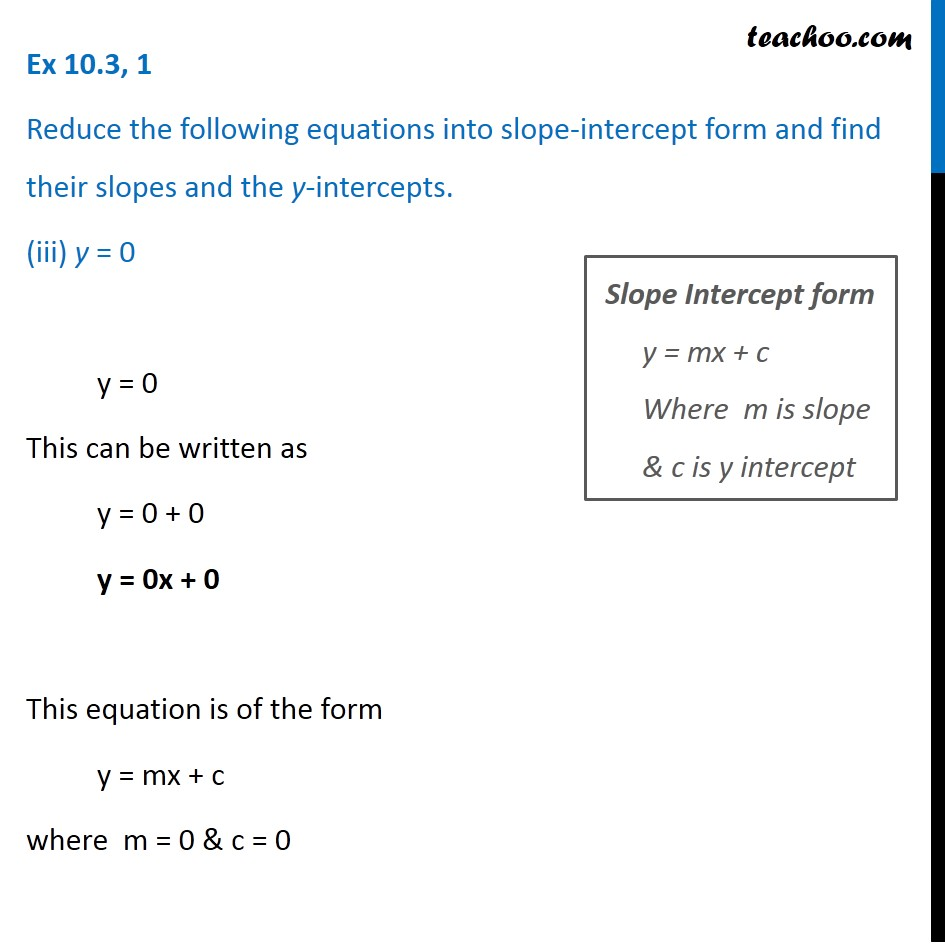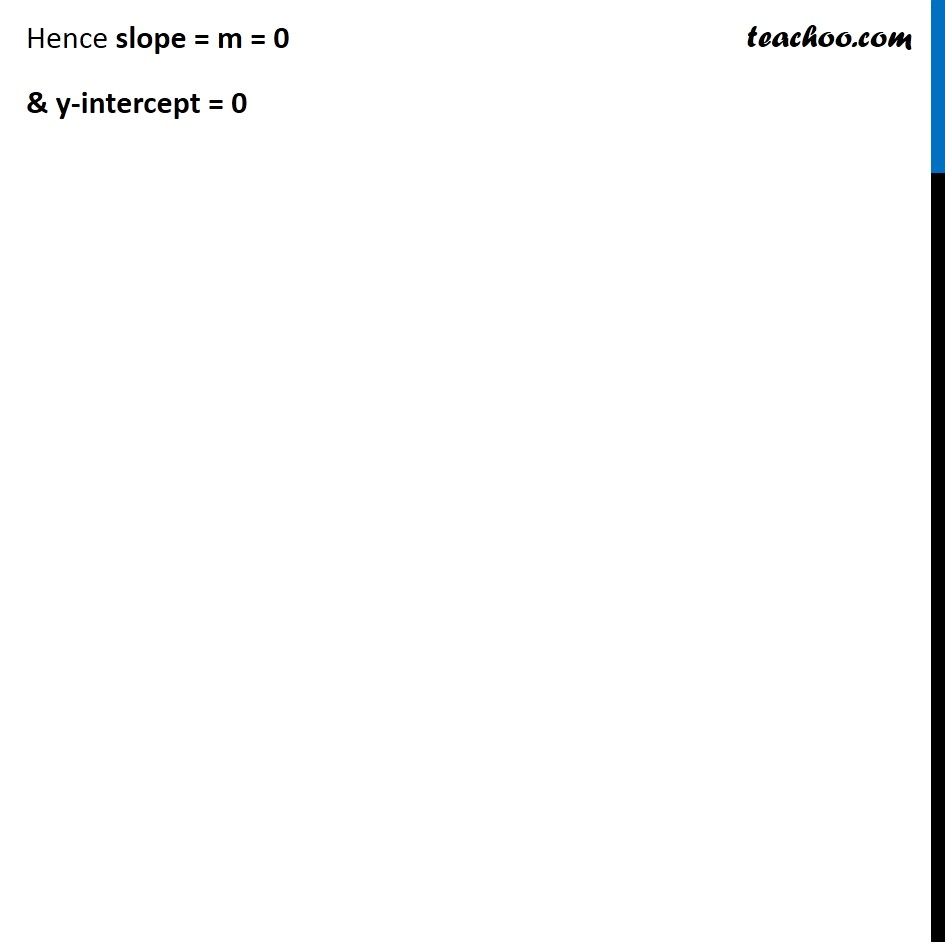Ex 9.3

Chapter 9 Class 11 Straight Lines
Serial order wiseLearn in your speed, with individual attention - Teachoo Maths 1-on-1 Class

### Transcript

Ex 9.3, 1 Reduce the following equations into slope-intercept form and find their slopes and the y-intercepts. (iii) y = 0 y = 0 This can be written as y = 0 + 0 y = 0x + 0 This equation is of the form y = mx + c where m = 0 & c = 0 Slope Intercept form y = mx + c Where m is slope & c is y intercept Hence slope = m = 0 & y-intercept = 0